Refraction of Waves

Refraction of Waves

Waves change direction when passing from one medium to another. This change in the direction of wave is called refraction of wave. During refraction velocity and wavelength of waves change however, frequency of waves stay constant. Velocity and wavelength of wave coming from deep part of water tank to shallow part decrease. Picture given below shows this change in the velocity and wavelength of waves.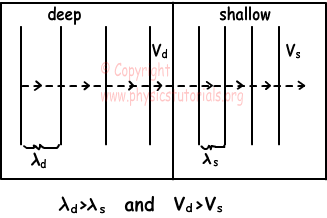If direction of waves coming from deep part of water tank is not perpendicular to the normal of the surface, then directions of refracted waves change. Look at the given picture below, it shows incident wave, refracted wave and angles between them.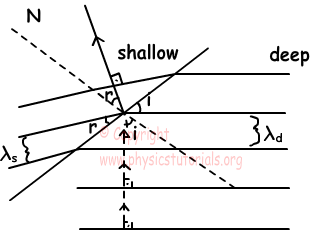We can write following equations for incident and refracted waves;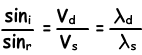Example: Velocity of the wave in the deep part of the tank is 16m/s. Picture given below shows the refraction of this wave when it pass to the shallow part of the tank. Find the wavelength of this wave in shallow part of the tank.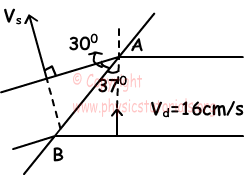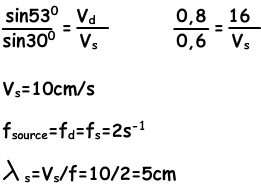Example: If the side view of the water tank is given in the picture below; draw the top view of the periodic linear waves produced by the source.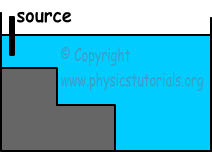There are  three different depths in this tank. Thus, velocity and wavelength of the deep part is bigger than the velocity and wavelength of the shallow part.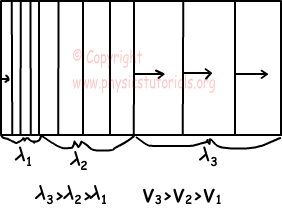Example: If the top view of the water tank is given below, find the shape of the linear wave after refracting from the deep part of the tank.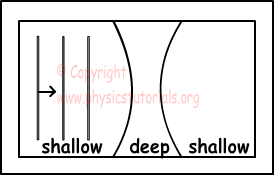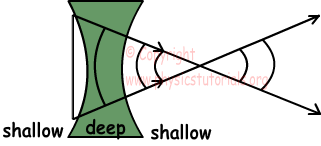First two ends of the linear wave enter to the deep water, so velocity of these points increase and linear wave becomes circular wave.

Waves Exams and Solutions

Author:

Tags: Waves Cheatsheet |